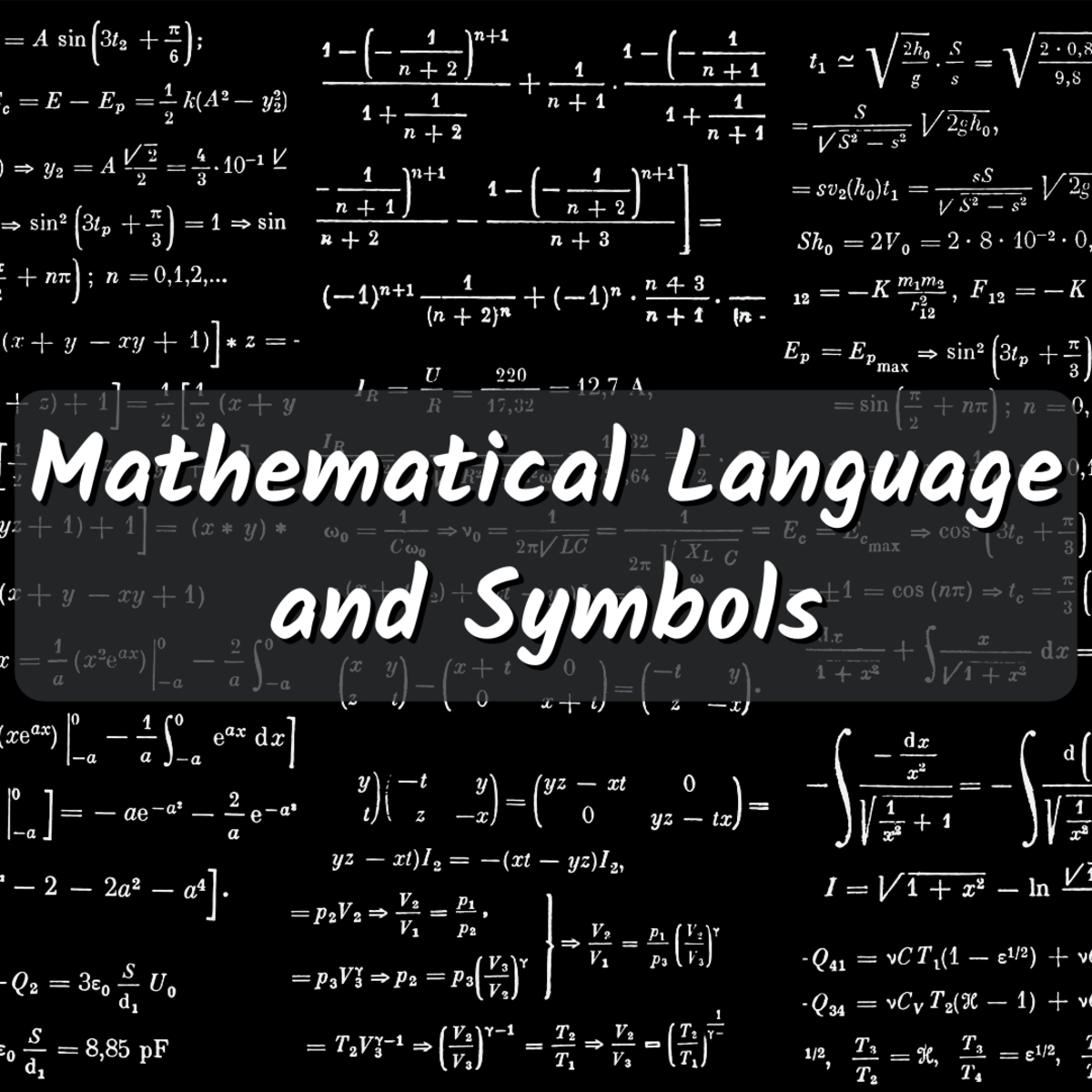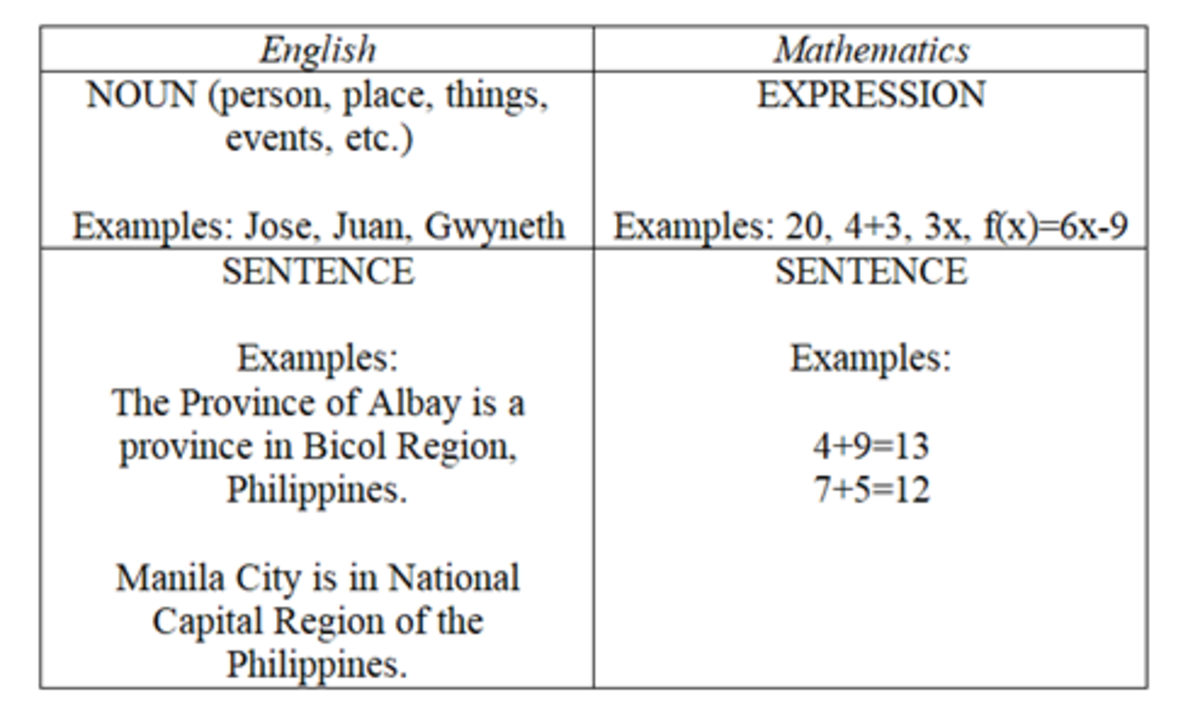# Mathematical Language and Symbols

Ryan Bernido is an educator and writer. He enjoys math and likes explaining it to his students and readers.Read on to learn all about mathematical language and symbols, including the difference between mathematical language and sentences, and the language of algebra.Dan-Cristian Pădureț | Unsplash

## Mathematical Language and Mathematical Symbols

Mathematical language is a system used in the field of mathematics to communicate mathematical ideas, concepts, and theories among people. It is distinct and unique from the usual language most people are used to and is used to communicate abstract, logical ideas.

Burns (n.d) mentioned three characteristics of mathematical language. These are being precise, being concise, and being powerful. Precision of mathematical language means the language is able to make very fine distinctions about and between things. Conciseness is the ability to say things briefly. Mathematical language being powerful is its ability to express complex thoughts with relative ease so that most people can understand them.

Mathematical symbols are used to refer to certain quantities, concepts, and ideas, among other things. The most commonly used symbols in basic mathematics are the numbers (0, 1, 2, 3, 4, 5, 6, 7, 8, 9), the four fundamental symbols of operation (+, -, ×, ÷), the inequality symbols (≠, ≤, ≥, < >), and the equal sign (=).

## Mathematical Expressions vs. Mathematical Sentences

A mathematical expression is the mathematical analogue of an English noun. That is, it is a correct arrangement of mathematical symbols used to represent a mathematical object of interest (Burns, n.d). It does not state a complete thought and it does not make sense to ask if an expression is true or false. In mathematics areas, such as Algebra, the most common expressions are numbers, sets, and functions.

A mathematical sentence is the mathematical analogue of an English sentence. That is, it is a correct arrangement of mathematical symbols that state a complete thought. Hence, it makes sense to ask if a sentence is true, false, sometimes true, or sometimes false.

Take a look at the table below to visualize the difference between expression and sentence in mathematics.This table visually demonstrates the difference between mathematical expressions and mathematical sentences.

## The Language of Algebra

Mathematical sentences and expressions are commonly used in Algebra. In fact, these concepts are generally taught to you during your 7th grade academic year. Let’s take a look back at the Language of Algebra where symbols, expressions, and mathematical sentences are first introduced.

### Common Words in Algebra

1. Variable – A symbol that represents a value or a number. Usually, small letters are used as variables. For instance, a, x, and y.
2. Constant – A number on its own, meaning the value is never definite and will never change. For instance, 4, 9, and 35.
3. Term – A constant, or a variable, or constant(s) and variable(s) multiplied together. For instance, 4, z, 5xy, 87xy, and xyz. The literal coefficient is the variable in a particular term. The numerical coefficient is the constant in a particular term.
4. Expression – Also called an algebraic expression, it is a group of terms separated by the “plus” or “minus” symbols. For instance, 3x-2 and x+3.

As mentioned, mathematics uses sentences. In Algebra, these sentences can be written mathematically or verbally. Mathematical sentences are written using variables, constants, and an equal sign or inequality sign. On the other hand, verbal sentences are written in words using phrases.

### Commonly Used Verbal Phrases in Algebra

1. Addition (“plus,” “increased by,” “added to,” “the sum of,” and “more than”)
2. Subtraction (“decreased by,” “subtracted from,” “the difference of,” “less than,” and “diminished by”)
3. Multiplication (“multiplied by,” “of,” “the product of,” and “times”)
4. Division (“ratio of,” “the quotient of,” and “divided by”)
5. Inequality (“is greater than,” “is less than,” “is at least,” “is less than or equal,” “is greater than or equal to,” “is at most,” and “is not equal to”)
6. Equality (“the same as,” “is equal to,” and “equals”)

This table gives you an idea of how you might translate various mathematical sentences into verbal sentences.

Mathematical SentenceVerbal Sentence

x + 2 = 12

The sum of a number x and two is equal to twelve.

9y = 18

Nine times a number y equals eighteen.

9 + 8 > 2

The sum of eight and nine is greater than one.

x – 8 ≤ 2

The difference of a number x and eight is less than or equal to two.

8x + 7 = 90

The sum of seven and eight times a number x is equal to ninety.

## Translating English to Math: Further Help With the Language of Algebra

This content is accurate and true to the best of the author’s knowledge and is not meant to substitute for formal and individualized advice from a qualified professional.

Scroll to Continue

Janine Vergara on September 28, 2020:

5/5 or 100%

Brian Angelo Dela Cruz on September 27, 2020:

5/5 or 100%

Patrick Pagal on September 27, 2020:

4/5 or 80%

Monica Jean Arango on September 27, 2020:

5/5 or 100% po

Joan Mae C. Bogñalbal on September 27, 2020:

5/5 or 100%

Christine B. Lucila on September 27, 2020:

5/5 or 100%

Clynt Justine O. Erasmo on September 27, 2020:

3/5 or 60%

Yeojie P. Mapula on September 26, 2020:

4/5 or 80%

Keith Renee Rayel on September 26, 2020:

5/5 or 100%

Rogyn P. Loquinario on September 26, 2020:

3/5 or 60 %

Ivy Mae T. Aranel on September 26, 2020:

5/5 or 100%

Ghienica D. Lucañas on September 25, 2020:

5/5 or 100%

Jamie Rose V. Manalo on September 25, 2020:

4/5 or 80%

Phoebe Maravilla on September 25, 2020:

5/5 or 100%

Paul Ago on September 25, 2020:

5/5 or 100%

Steve Dolz on September 25, 2020:

5/5 or 100%

Janine A. De la Rama on September 25, 2020:

5/5 or 100%

John Edward G. Bobier on September 25, 2020:

5/5 or 100%

Kiarra Queen Bragais on September 24, 2020:

5/5 or 100%

Angela Naris on September 24, 2020:

5/5(100%)

Criselle Morano on September 24, 2020:

4/5 (80%)

Kyla Victoria on September 24, 2020:

5/5 or 100%

Angelica Panique on September 24, 2020:

4/5 80%

Ericka Kaye Gison on September 24, 2020:

5/5 or 100%

Crizielle Reniva on September 24, 2020:

100%

Ma. Sophia Ajero on September 24, 2020:

5/5 100%

Ellyzabelle Louise Yu Don on September 24, 2020:

5/5 = 100%

Ma. Christine S. Moreno on September 24, 2020:

I got 100% score.